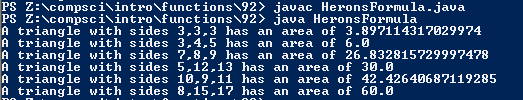# Assignment #92

## Code

```    /// Name: Ian Stanko
/// Period: 5
/// Program Name: HeronsFormula
/// File Name: HeronsFormula.java
/// Date Finished: 3/5/2016

public class HeronsFormula
{
public static void main( String[] args )
{
double a;

a = triangleArea(3, 3, 3);
System.out.println("A triangle with sides 3,3,3 has an area of " + a );

a = triangleArea(3, 4, 5);
System.out.println("A triangle with sides 3,4,5 has an area of " + a );

a = triangleArea(7, 8, 9);
System.out.println("A triangle with sides 7,8,9 has an area of " + a );

System.out.println("A triangle with sides 5,12,13 has an area of " + triangleArea(5, 12, 13) );
System.out.println("A triangle with sides 10,9,11 has an area of " + triangleArea(10, 9, 11) );
System.out.println("A triangle with sides 8,15,17 has an area of " + triangleArea(8, 15, 17) );
}

public static double triangleArea( int a, int b, int c )
{
// the code in this function computes the area of a triangle whose sides have lengths a, b, and c
double s, A;

s = (a+b+c) / 2.0;
A = Math.sqrt( s*(s-a)*(s-b)*(s-c) );

return A;
// ^ after computing the area, "return" it
}
}
///Both the programs return the same thing
///The HeronsFormulaNofunction has 57 lines of code, whereas the HeronsFormula prgram has 36 lines
///It was esaier to change the code with the function becuase it reuses the code instead of rewriting it several times

```

### Picture of the output HOME